Скачать презентацию REDUCING ELECTRICAL ENERGY CONSUMPTION Lesson 13 A November

eb658395fdf1c138026b182df7746523.ppt

• Количество слайдов: 25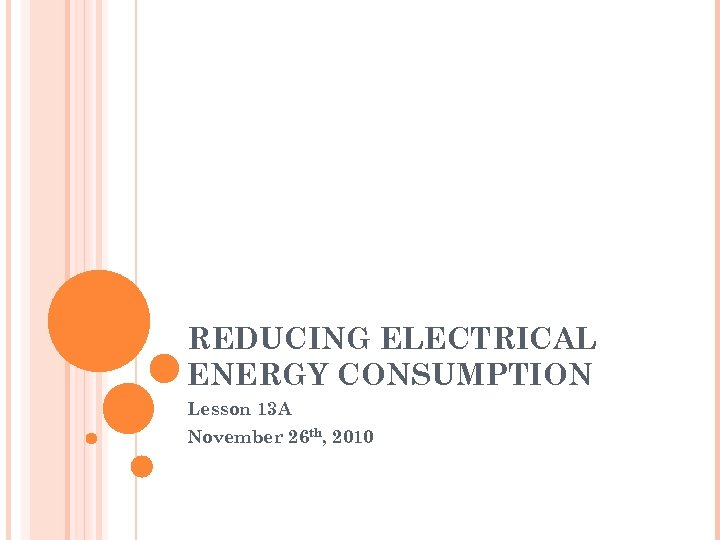REDUCING ELECTRICAL ENERGY CONSUMPTION Lesson 13 A November 26 th, 2010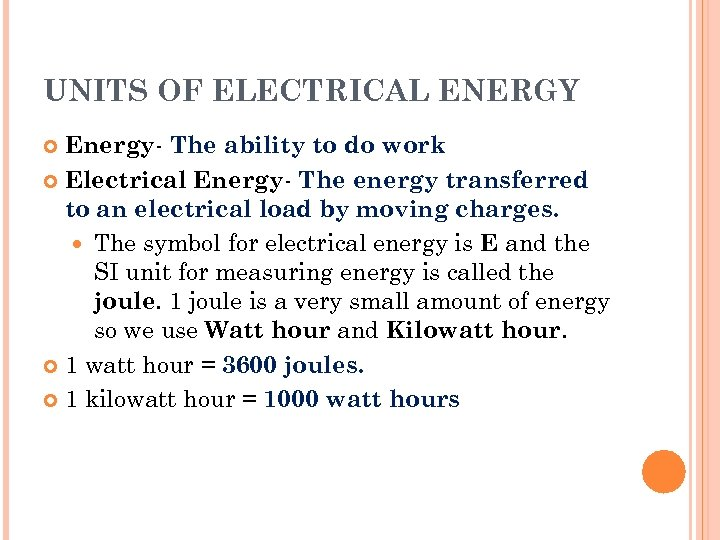UNITS OF ELECTRICAL ENERGY Energy- The ability to do work Electrical Energy- The energy transferred to an electrical load by moving charges. The symbol for electrical energy is E and the SI unit for measuring energy is called the joule. 1 joule is a very small amount of energy so we use Watt hour and Kilowatt hour. 1 watt hour = 3600 joules. 1 kilowatt hour = 1000 watt hours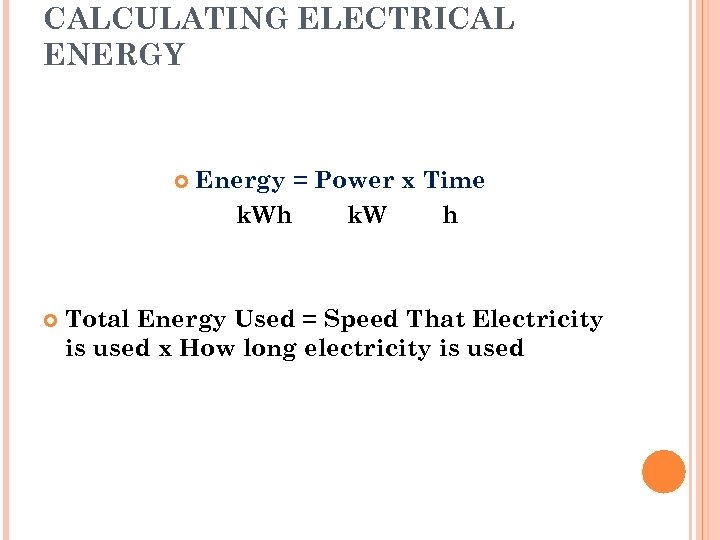CALCULATING ELECTRICAL ENERGY Energy = Power x Time k. Wh k. W h Total Energy Used = Speed That Electricity is used x How long electricity is used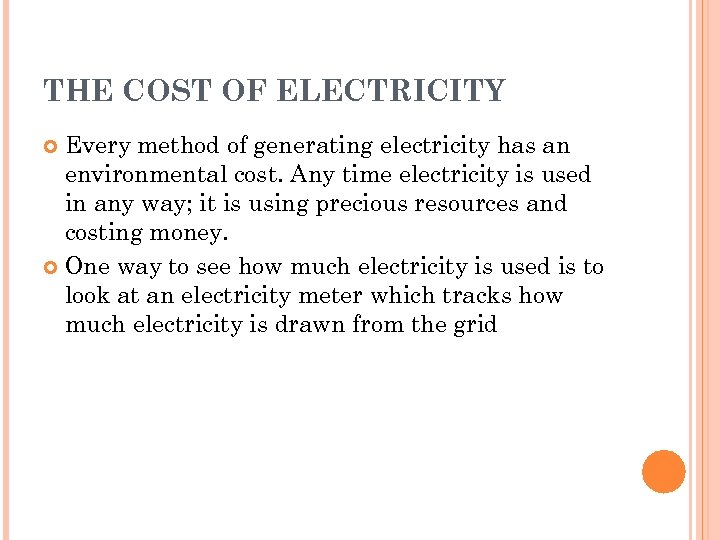THE COST OF ELECTRICITY Every method of generating electricity has an environmental cost. Any time electricity is used in any way; it is using precious resources and costing money. One way to see how much electricity is used is to look at an electricity meter which tracks how much electricity is drawn from the grid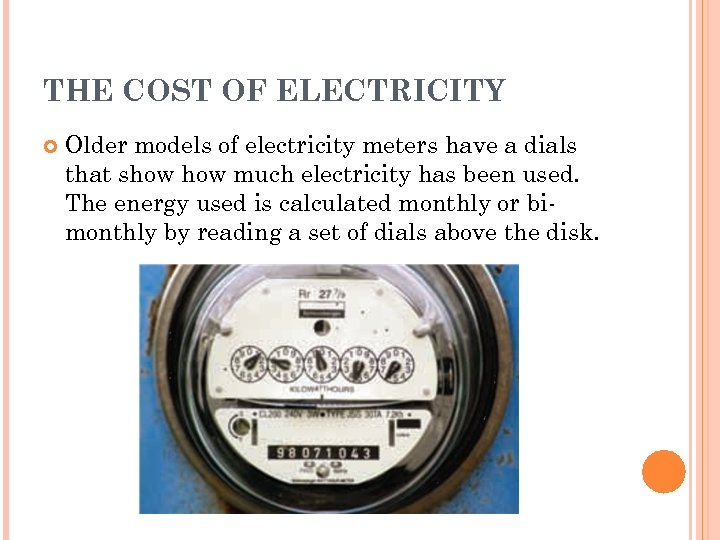THE COST OF ELECTRICITY Older models of electricity meters have a dials that show much electricity has been used. The energy used is calculated monthly or bimonthly by reading a set of dials above the disk.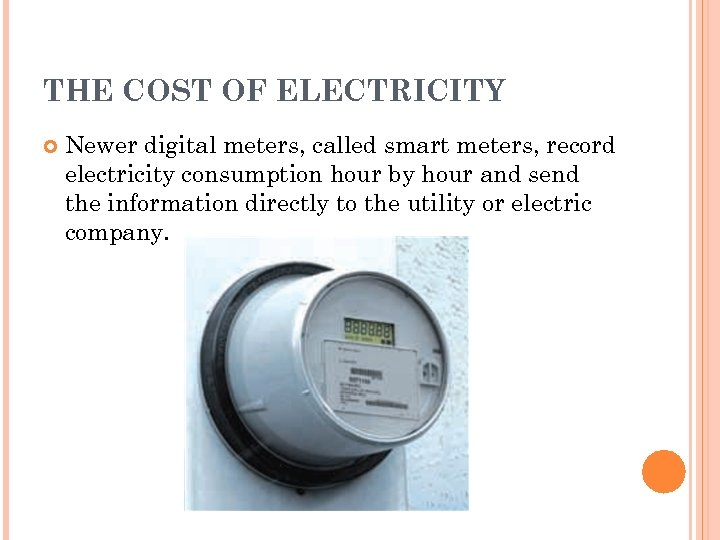THE COST OF ELECTRICITY Newer digital meters, called smart meters, record electricity consumption hour by hour and send the information directly to the utility or electric company.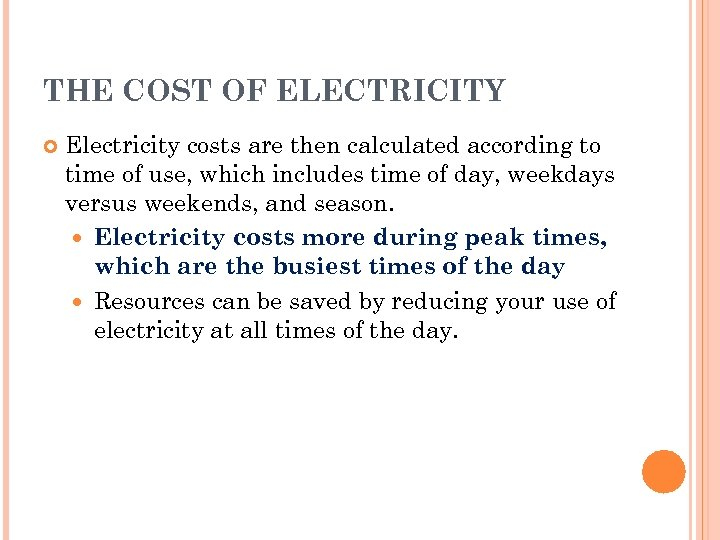THE COST OF ELECTRICITY Electricity costs are then calculated according to time of use, which includes time of day, weekdays versus weekends, and season. Electricity costs more during peak times, which are the busiest times of the day Resources can be saved by reducing your use of electricity at all times of the day.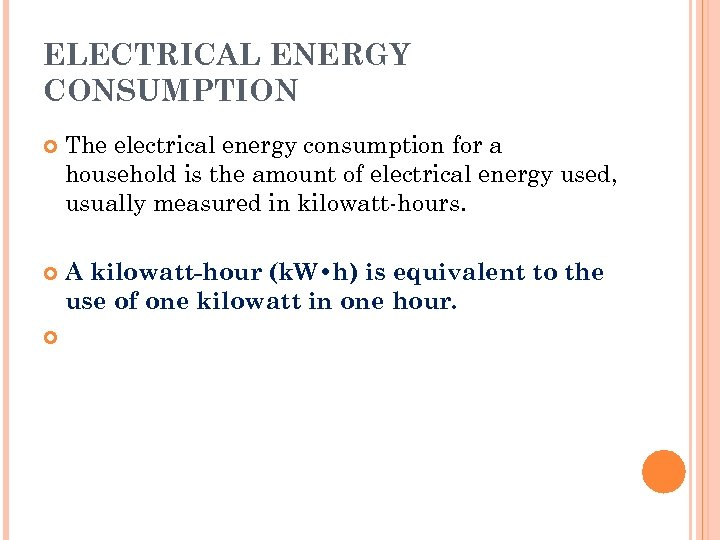ELECTRICAL ENERGY CONSUMPTION The electrical energy consumption for a household is the amount of electrical energy used, usually measured in kilowatt-hours. A kilowatt-hour (k. W • h) is equivalent to the use of one kilowatt in one hour.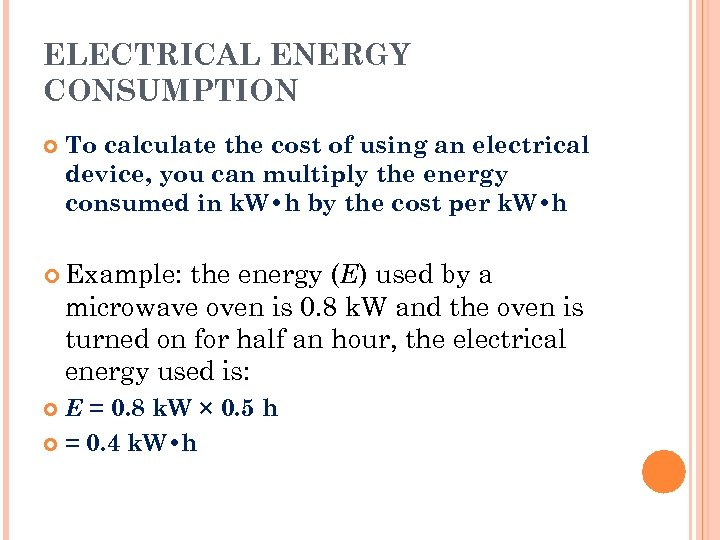ELECTRICAL ENERGY CONSUMPTION To calculate the cost of using an electrical device, you can multiply the energy consumed in k. W • h by the cost per k. W • h Example: the energy (E) used by a microwave oven is 0. 8 k. W and the oven is turned on for half an hour, the electrical energy used is: E = 0. 8 k. W × 0. 5 h = 0. 4 k. W • h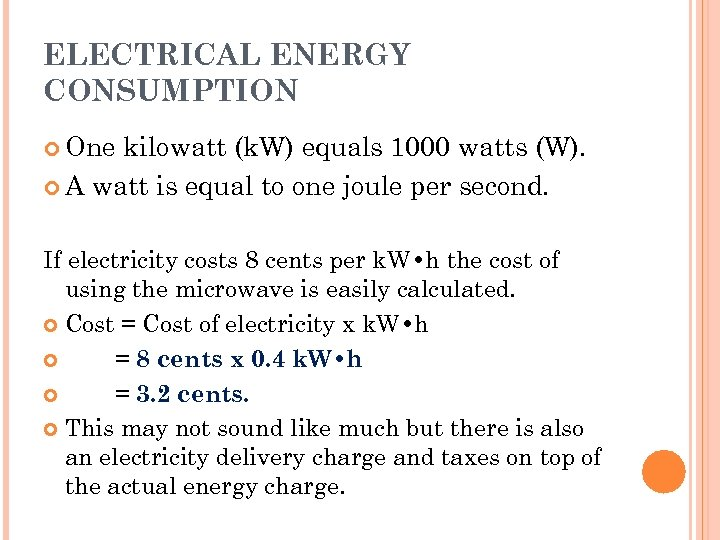ELECTRICAL ENERGY CONSUMPTION One kilowatt (k. W) equals 1000 watts (W). A watt is equal to one joule per second. If electricity costs 8 cents per k. W • h the cost of using the microwave is easily calculated. Cost = Cost of electricity x k. W • h = 8 cents x 0. 4 k. W • h = 3. 2 cents. This may not sound like much but there is also an electricity delivery charge and taxes on top of the actual energy charge.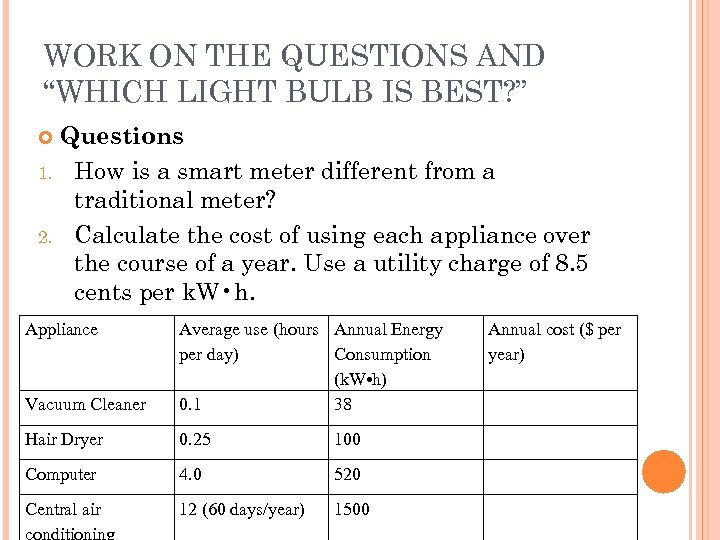WORK ON THE QUESTIONS AND “WHICH LIGHT BULB IS BEST? ” Questions 1. How is a smart meter different from a traditional meter? 2. Calculate the cost of using each appliance over the course of a year. Use a utility charge of 8. 5 cents per k. W • h. Appliance Vacuum Cleaner Average use (hours Annual Energy per day) Consumption (k. W • h) 0. 1 38 Hair Dryer 0. 25 100 Computer 4. 0 520 Central air conditioning 12 (60 days/year) 1500 Annual cost (\$ per year)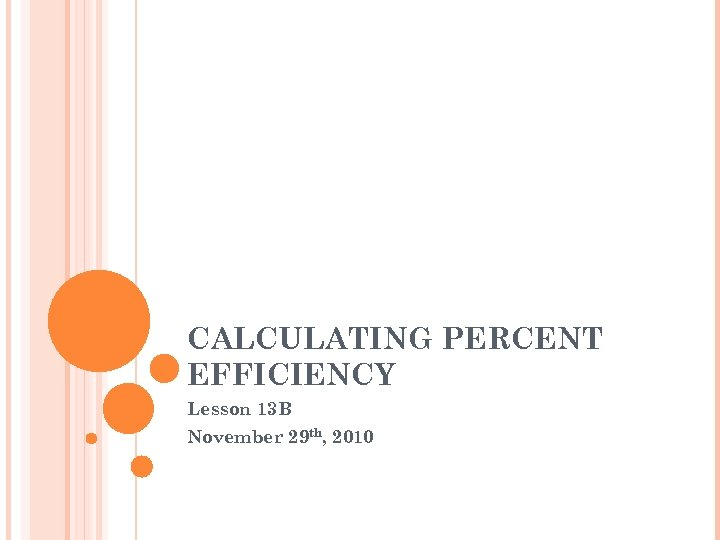CALCULATING PERCENT EFFICIENCY Lesson 13 B November 29 th, 2010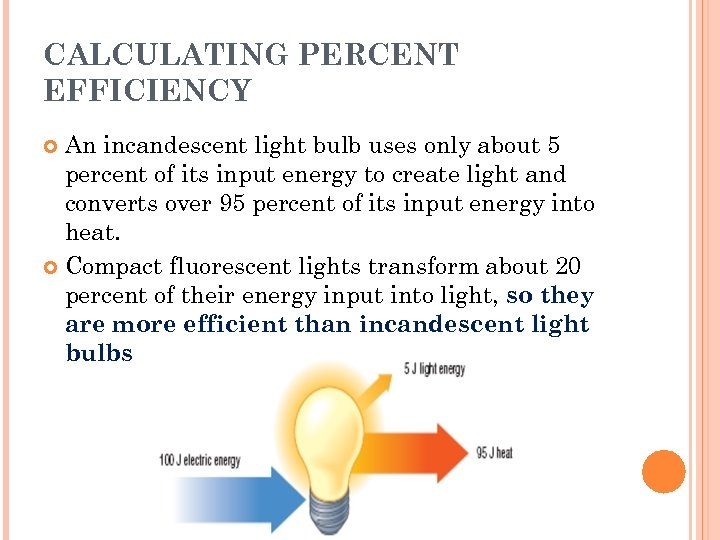CALCULATING PERCENT EFFICIENCY An incandescent light bulb uses only about 5 percent of its input energy to create light and converts over 95 percent of its input energy into heat. Compact fluorescent lights transform about 20 percent of their energy input into light, so they are more efficient than incandescent light bulbs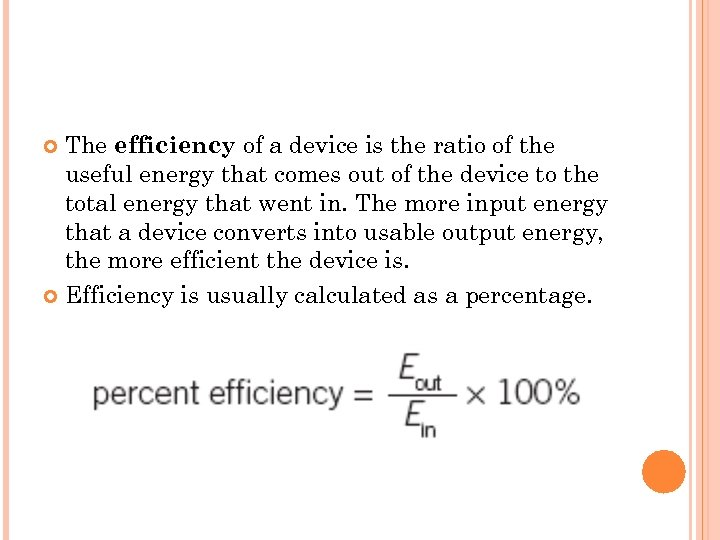The efficiency of a device is the ratio of the useful energy that comes out of the device to the total energy that went in. The more input energy that a device converts into usable output energy, the more efficient the device is. Efficiency is usually calculated as a percentage.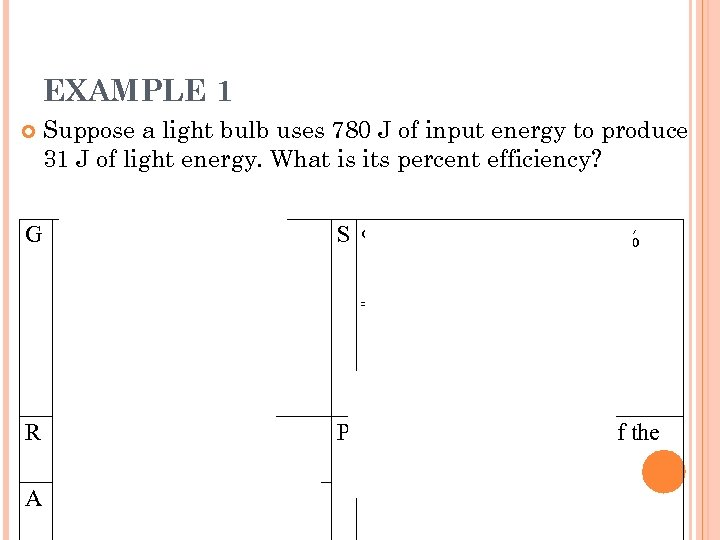EXAMPLE 1 Suppose a light bulb uses 780 J of input energy to produce 31 J of light energy. What is its percent efficiency? G Input energy = 780 J Output energy = 31 J S % efficiency = 31 J x 100% 780 J = 4. 0 % R Percent efficiency = ? P Therefore, the efficiency of the light bulb is 4. 0 percent. A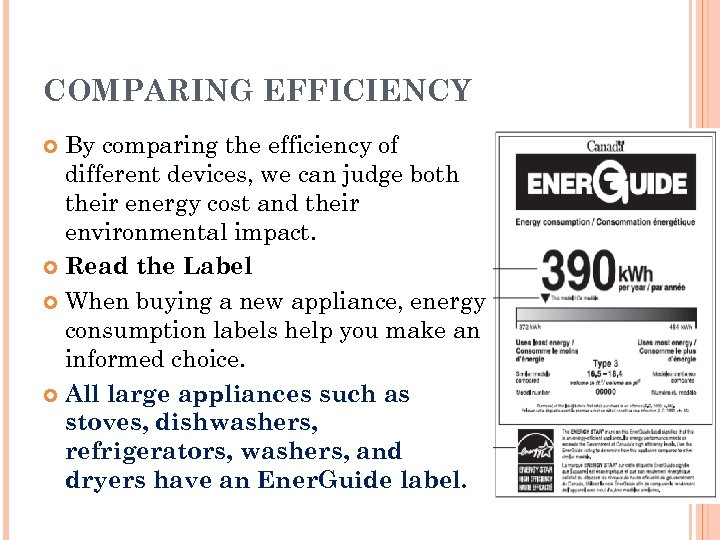COMPARING EFFICIENCY By comparing the efficiency of different devices, we can judge both their energy cost and their environmental impact. Read the Label When buying a new appliance, energy consumption labels help you make an informed choice. All large appliances such as stoves, dishwashers, refrigerators, washers, and dryers have an Ener. Guide label.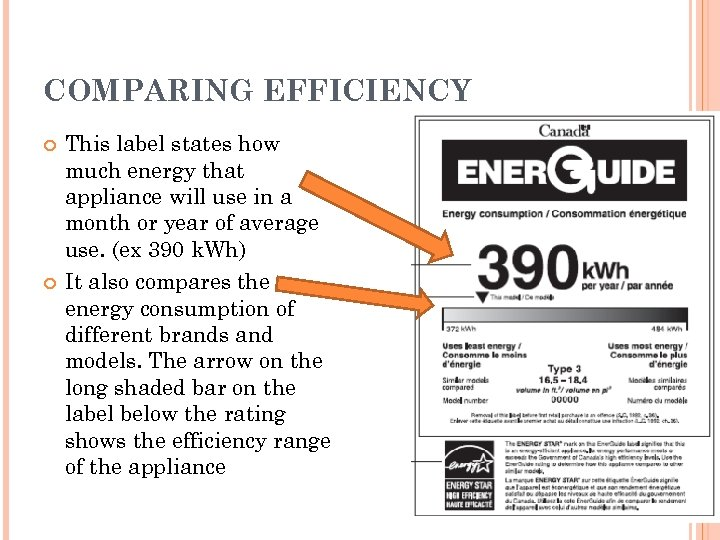COMPARING EFFICIENCY This label states how much energy that appliance will use in a month or year of average use. (ex 390 k. Wh) It also compares the energy consumption of different brands and models. The arrow on the long shaded bar on the label below the rating shows the efficiency range of the appliance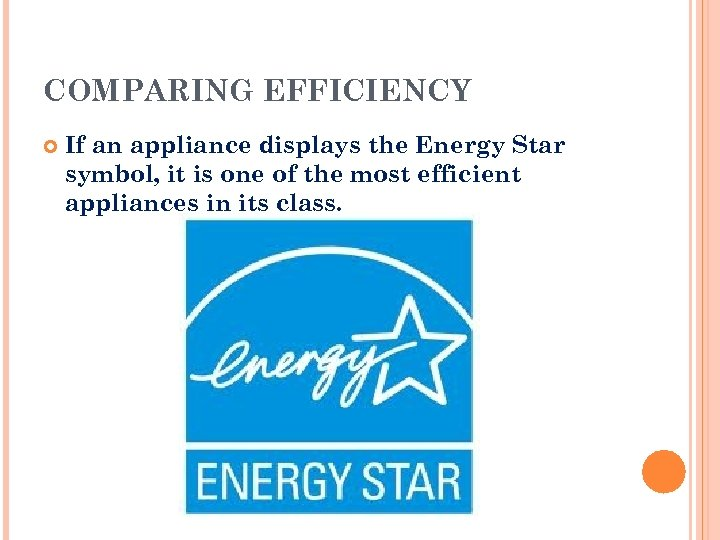COMPARING EFFICIENCY If an appliance displays the Energy Star symbol, it is one of the most efficient appliances in its class.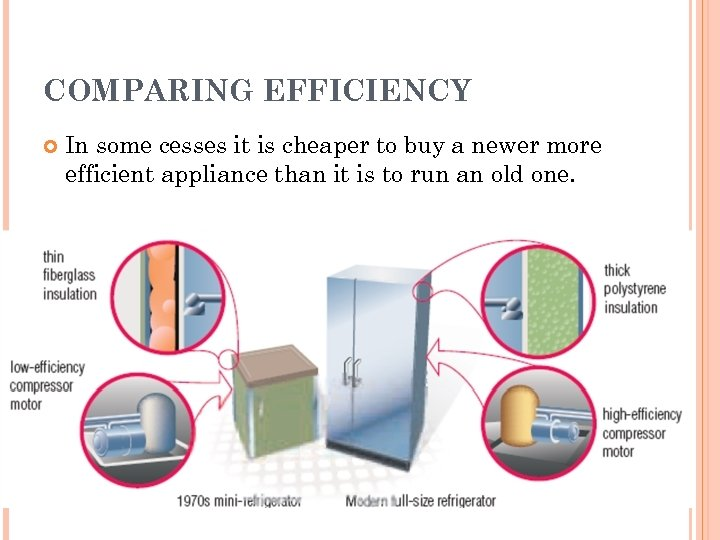COMPARING EFFICIENCY In some cesses it is cheaper to buy a newer more efficient appliance than it is to run an old one.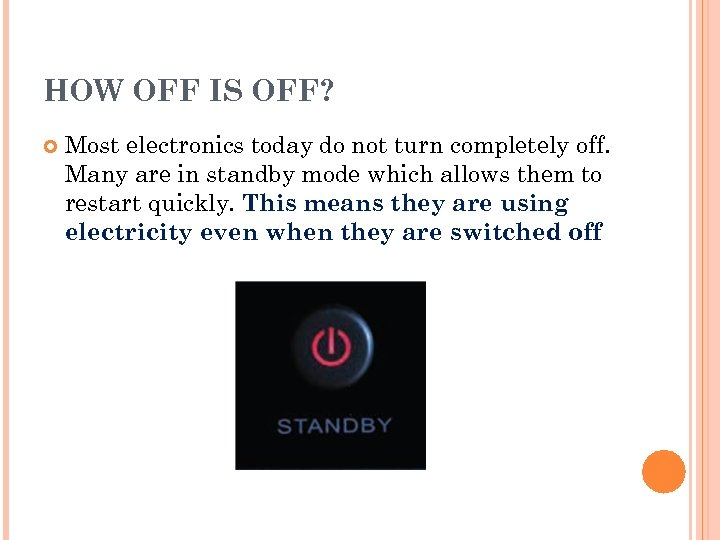HOW OFF IS OFF? Most electronics today do not turn completely off. Many are in standby mode which allows them to restart quickly. This means they are using electricity even when they are switched off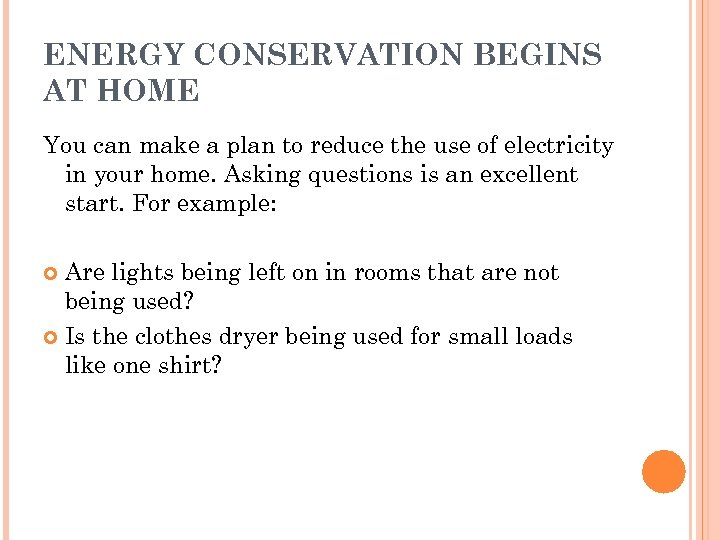ENERGY CONSERVATION BEGINS AT HOME You can make a plan to reduce the use of electricity in your home. Asking questions is an excellent start. For example: Are lights being left on in rooms that are not being used? Is the clothes dryer being used for small loads like one shirt?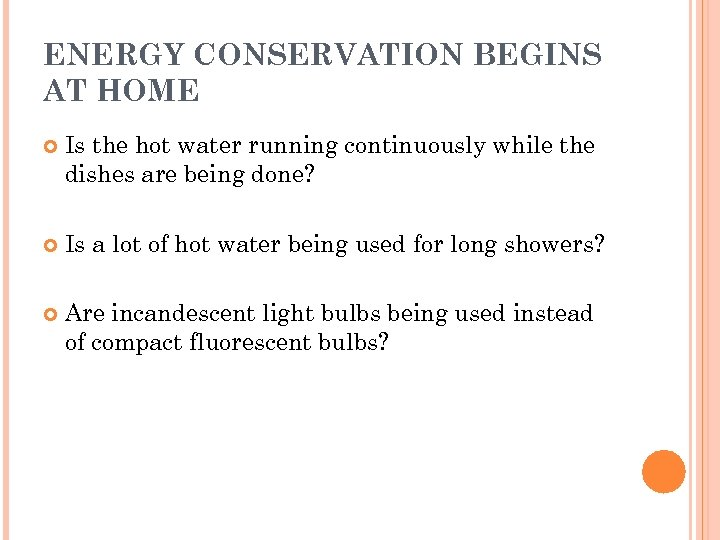ENERGY CONSERVATION BEGINS AT HOME Is the hot water running continuously while the dishes are being done? Is a lot of hot water being used for long showers? Are incandescent light bulbs being used instead of compact fluorescent bulbs?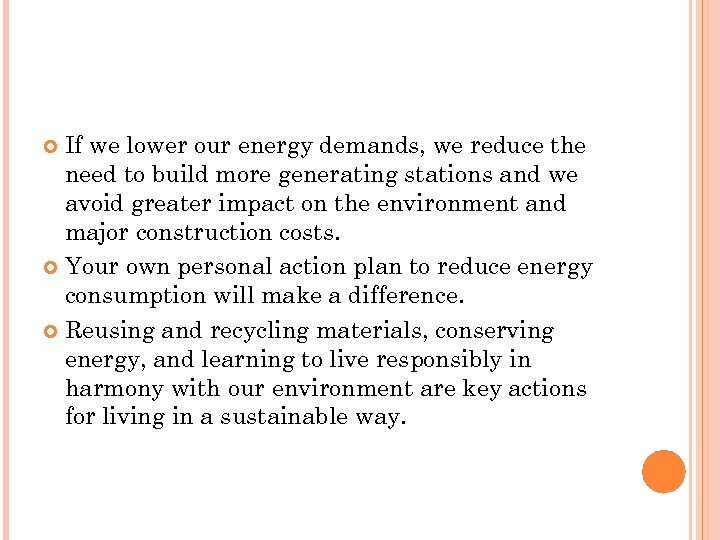If we lower our energy demands, we reduce the need to build more generating stations and we avoid greater impact on the environment and major construction costs. Your own personal action plan to reduce energy consumption will make a difference. Reusing and recycling materials, conserving energy, and learning to live responsibly in harmony with our environment are key actions for living in a sustainable way.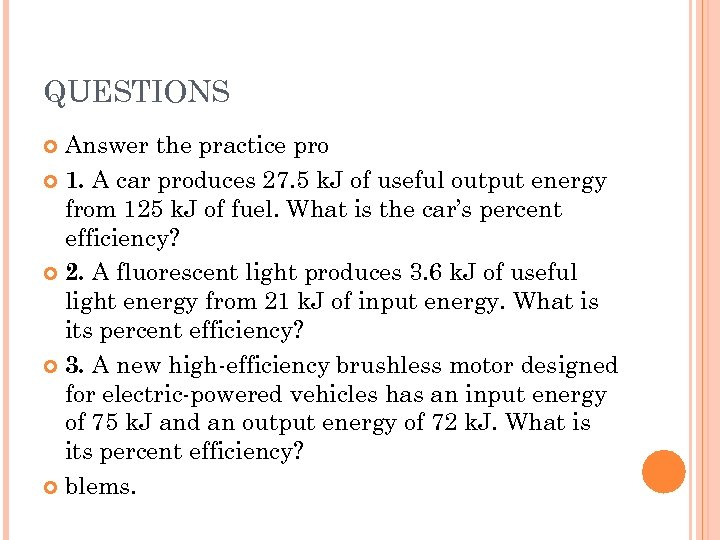QUESTIONS Answer the practice pro 1. A car produces 27. 5 k. J of useful output energy from 125 k. J of fuel. What is the car’s percent efficiency? 2. A fluorescent light produces 3. 6 k. J of useful light energy from 21 k. J of input energy. What is its percent efficiency? 3. A new high-efficiency brushless motor designed for electric-powered vehicles has an input energy of 75 k. J and an output energy of 72 k. J. What is its percent efficiency? blems.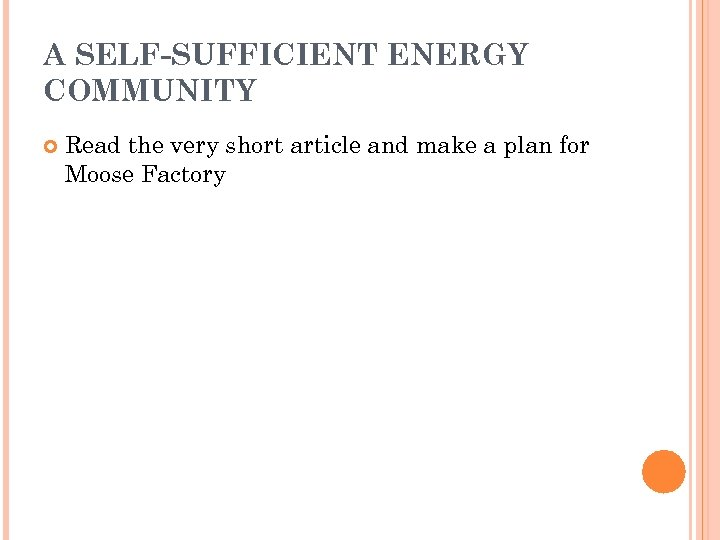A SELF-SUFFICIENT ENERGY COMMUNITY Read the very short article and make a plan for Moose Factory